#Separable EquationsThe differential equation of the form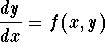is called separable, if f(x,y) = h(x) g(y); that is,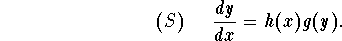In order to solve it, perform the following steps:

(1)
Solve the equation g(y) = 0, which gives the constant solutions of (S);
(2)
Rewrite the equation (S) as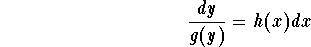,

and, then, integrate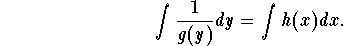to obtain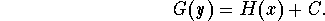(3)
Write down all the solutions; the constant ones obtained from (1) and the ones given in (2);
(4)
If you are given an IVP, use the initial condition to find the particular solution. Note that it may happen that the particular solution is one of the constant solutions given in (1). This is why Step 3 is important.

Example: Find the particular solution of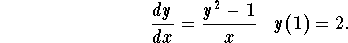Solution: Perform the following steps:

(1)
In order to find the constant solutions, solve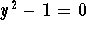. We obtain y = 1 and y=-1.
(2)
Rewrite the equation as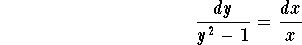.

Using the techniques of integration of rational functions, we get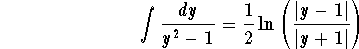,

which implies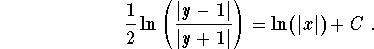(3)
The solutions to the given differential equation are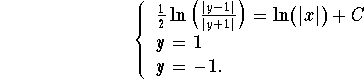(4)
Since the constant solutions do not satisfy the initial condition, we are left to find the particular solution among the ones found in (2), that is we need to find the constant C. If we plug in the condition y=2 when x=1, we get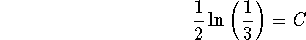.

Note that this solution is given in an implicit form. You may be asked to rewrite it in an explicit one. For example, in this case, we have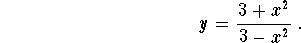### If you would like more practice, click on Example.[Differential Equations] [Linear Equations] [Next Example]
[Geometry] [Algebra] [Trigonometry ]
[Calculus] [Complex Variables] [Matrix Algebra]S.O.S. MATHematics home page

Do you need more help? Please post your question on our S.O.S. Mathematics CyberBoard.Author: Mohamed Amine Khamsi
Last Update 6-22-98